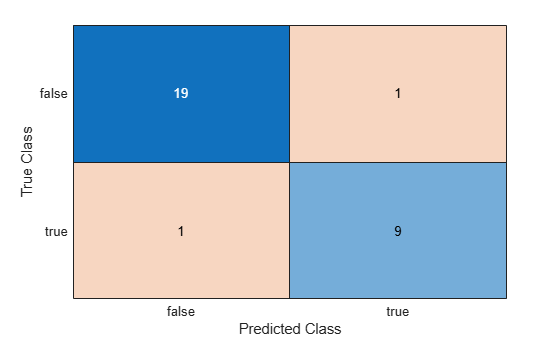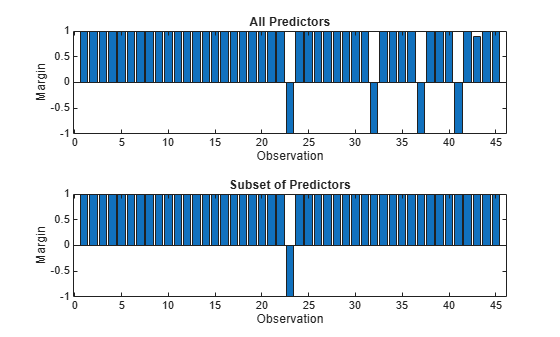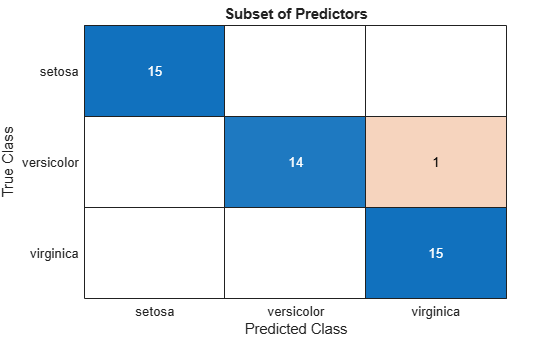# predict

Classify observations using neural network classifier

Since R2021a

## Syntax

``label = predict(Mdl,X)``
``label = predict(Mdl,X,'ObservationsIn',dimension)``
``[label,Score] = predict(___)``

## Description

example

````label = predict(Mdl,X)` returns predicted class labels for the predictor data in the table or matrix `X` using the trained neural network classification model `Mdl`.```
````label = predict(Mdl,X,'ObservationsIn',dimension)` specifies the predictor data observation dimension, either `'rows'` (default) or `'columns'`. For example, specify `'ObservationsIn','columns'` to indicate that columns in the predictor data correspond to observations.```

example

````[label,Score] = predict(___)` also returns a matrix of classification scores indicating the likelihood that a label comes from a particular class, using any of the input argument combinations in the previous syntaxes. For each observation in `X`, the predicted class label corresponds to the maximum score among all classes.```

## Examples

collapse all

Predict labels for test set observations using a neural network classifier.

Load the `patients` data set. Create a table from the data set. Each row corresponds to one patient, and each column corresponds to a diagnostic variable. Use the `Smoker` variable as the response variable, and the rest of the variables as predictors.

```load patients tbl = table(Diastolic,Systolic,Gender,Height,Weight,Age,Smoker);```

Separate the data into a training set `tblTrain` and a test set `tblTest` by using a stratified holdout partition. The software reserves approximately 30% of the observations for the test data set and uses the rest of the observations for the training data set.

```rng("default") % For reproducibility of the partition c = cvpartition(tbl.Smoker,"Holdout",0.30); trainingIndices = training(c); testIndices = test(c); tblTrain = tbl(trainingIndices,:); tblTest = tbl(testIndices,:);```

Train a neural network classifier using the training set. Specify the `Smoker` column of `tblTrain` as the response variable. Specify to standardize the numeric predictors.

```Mdl = fitcnet(tblTrain,"Smoker", ... "Standardize",true);```

Classify the test set observations. Visualize the results using a confusion matrix.

```label = predict(Mdl,tblTest); confusionchart(tblTest.Smoker,label)```The neural network model correctly classifies all but two of the test set observations.

Perform feature selection by comparing test set classification margins, edges, errors, and predictions. Compare the test set metrics for a model trained using all the predictors to the test set metrics for a model trained using only a subset of the predictors.

Load the sample file `fisheriris.csv`, which contains iris data including sepal length, sepal width, petal length, petal width, and species type. Read the file into a table.

`fishertable = readtable('fisheriris.csv');`

Separate the data into a training set `trainTbl` and a test set `testTbl` by using a stratified holdout partition. The software reserves approximately 30% of the observations for the test data set and uses the rest of the observations for the training data set.

```rng("default") c = cvpartition(fishertable.Species,"Holdout",0.3); trainTbl = fishertable(training(c),:); testTbl = fishertable(test(c),:);```

Train one neural network classifier using all the predictors in the training set, and train another classifier using all the predictors except `PetalWidth`. For both models, specify `Species` as the response variable, and standardize the predictors.

```allMdl = fitcnet(trainTbl,"Species","Standardize",true); subsetMdl = fitcnet(trainTbl,"Species ~ SepalLength + SepalWidth + PetalLength", ... "Standardize",true);```

Calculate the test set classification margins for the two models. Because the test set includes only 45 observations, display the margins using bar graphs.

For each observation, the classification margin is the difference between the classification score for the true class and the maximal score for the false classes. Because neural network classifiers return classification scores that are posterior probabilities, margin values close to 1 indicate confident classifications and negative margin values indicate misclassifications.

```tiledlayout(2,1) % Top axes ax1 = nexttile; allMargins = margin(allMdl,testTbl); bar(ax1,allMargins) xlabel(ax1,"Observation") ylabel(ax1,"Margin") title(ax1,"All Predictors") % Bottom axes ax2 = nexttile; subsetMargins = margin(subsetMdl,testTbl); bar(ax2,subsetMargins) xlabel(ax2,"Observation") ylabel(ax2,"Margin") title(ax2,"Subset of Predictors")```Compare the test set classification edge, or mean of the classification margins, of the two models.

`allEdge = edge(allMdl,testTbl)`
```allEdge = 0.8198 ```
`subsetEdge = edge(subsetMdl,testTbl)`
```subsetEdge = 0.9556 ```

Based on the test set classification margins and edges, the model trained on a subset of the predictors seems to outperform the model trained on all the predictors.

Compare the test set classification error of the two models.

```allError = loss(allMdl,testTbl); allAccuracy = 1-allError```
```allAccuracy = 0.9111 ```
```subsetError = loss(subsetMdl,testTbl); subsetAccuracy = 1-subsetError```
```subsetAccuracy = 0.9778 ```

Again, the model trained using only a subset of the predictors seems to perform better than the model trained using all the predictors.

Visualize the test set classification results using confusion matrices.

```allLabels = predict(allMdl,testTbl); figure confusionchart(testTbl.Species,allLabels) title("All Predictors")``````subsetLabels = predict(subsetMdl,testTbl); figure confusionchart(testTbl.Species,subsetLabels) title("Subset of Predictors")```The model trained using all the predictors misclassifies four of the test set observations. The model trained using a subset of the predictors misclassifies only one of the test set observations.

Given the test set performance of the two models, consider using the model trained using all the predictors except `PetalWidth`.

See how the layers of a neural network classifier work together to predict the label and classification scores for a single observation.

Load the sample file `fisheriris.csv`, which contains iris data including sepal length, sepal width, petal length, petal width, and species type. Read the file into a table.

`fishertable = readtable('fisheriris.csv');`

Train a neural network classifier using the data set. Specify the `Species` column of `fishertable` as the response variable.

`Mdl = fitcnet(fishertable,"Species");`

Select the fifteenth observation from the data set. See how the layers of the neural network classifier take the observation and return a predicted class label `newPointLabel` and classification scores `newPointScores`.

`newPoint = Mdl.X{15,:}`
```newPoint = 1×4 5.8000 4.0000 1.2000 0.2000 ```
```firstFCStep = (Mdl.LayerWeights{1})*newPoint' + Mdl.LayerBiases{1}; reluStep = max(firstFCStep,0); finalFCStep = (Mdl.LayerWeights{end})*reluStep + Mdl.LayerBiases{end}; finalSoftmaxStep = softmax(finalFCStep); [~,classIdx] = max(finalSoftmaxStep); newPointLabel = Mdl.ClassNames{classIdx}```
```newPointLabel = 'setosa' ```
`newPointScores = finalSoftmaxStep'`
```newPointScores = 1×3 1.0000 0.0000 0.0000 ```

Check that the predictions match those returned by the `predict` object function.

`[predictedLabel,predictedScores] = predict(Mdl,newPoint)`
```predictedLabel = 1x1 cell array {'setosa'} ```
```predictedScores = 1×3 1.0000 0.0000 0.0000 ```

## Input Arguments

collapse all

Trained neural network classifier, specified as a `ClassificationNeuralNetwork` model object or `CompactClassificationNeuralNetwork` model object returned by `fitcnet` or `compact`, respectively.

Predictor data to be classified, specified as a numeric matrix or table.

By default, each row of `X` corresponds to one observation, and each column corresponds to one variable.

• For a numeric matrix:

• The variables in the columns of `X` must have the same order as the predictor variables that trained `Mdl`.

• If you train `Mdl` using a table (for example, `Tbl`) and `Tbl` contains only numeric predictor variables, then `X` can be a numeric matrix. To treat numeric predictors in `Tbl` as categorical during training, identify categorical predictors by using the `CategoricalPredictors` name-value argument of `fitcnet`. If `Tbl` contains heterogeneous predictor variables (for example, numeric and categorical data types) and `X` is a numeric matrix, then `predict` throws an error.

• For a table:

• `predict` does not support multicolumn variables or cell arrays other than cell arrays of character vectors.

• If you train `Mdl` using a table (for example, `Tbl`), then all predictor variables in `X` must have the same variable names and data types as the variables that trained `Mdl` (stored in `Mdl.PredictorNames`). However, the column order of `X` does not need to correspond to the column order of `Tbl`. Also, `Tbl` and `X` can contain additional variables (response variables, observation weights, and so on), but `predict` ignores them.

• If you train `Mdl` using a numeric matrix, then the predictor names in `Mdl.PredictorNames` must be the same as the corresponding predictor variable names in `X`. To specify predictor names during training, use the `PredictorNames` name-value argument of `fitcnet`. All predictor variables in `X` must be numeric vectors. `X` can contain additional variables (response variables, observation weights, and so on), but `predict` ignores them.

If you set `'Standardize',true` in `fitcnet` when training `Mdl`, then the software standardizes the numeric columns of the predictor data using the corresponding means and standard deviations.

Note

If you orient your predictor matrix so that observations correspond to columns and specify `'ObservationsIn','columns'`, then you might experience a significant reduction in computation time. You cannot specify `'ObservationsIn','columns'` for predictor data in a table.

Data Types: `single` | `double` | `table`

Predictor data observation dimension, specified as `'rows'` or `'columns'`.

Note

If you orient your predictor matrix so that observations correspond to columns and specify `'ObservationsIn','columns'`, then you might experience a significant reduction in computation time. You cannot specify `'ObservationsIn','columns'` for predictor data in a table.

Data Types: `char` | `string`

## Output Arguments

collapse all

Predicted class labels, returned as a numeric, categorical, or logical vector; a character or string array; or a cell array of character vectors. The software predicts the classification of an observation by assigning the observation to the class yielding the largest classification score or posterior probability.

`label` has the same data type as the observed class labels that trained `Mdl`, and its length is equal to the number of observations in `X`. (The software treats string arrays as cell arrays of character vectors.)

Classification scores, returned as an n-by-K matrix, where n is the number of observations in `X` and K is the number of unique classes. The classification score `Score(i,j)` represents the posterior probability that the `i`th observation belongs to class `j`.

collapse all

### Classification Scores

The classification scores for a neural network classifier are computed using the softmax activation function that follows the final fully connected layer in the network. The scores correspond to posterior probabilities.

The posterior probability that an observation x is of class k is

`$\stackrel{^}{P}\left(k|x\right)=\frac{P\left(x|k\right)P\left(k\right)}{\sum _{j=1}^{K}P\left(x|j\right)P\left(j\right)}=\frac{\mathrm{exp}\left({a}_{k}\left(x\right)\right)}{\sum _{j=1}^{K}\mathrm{exp}\left({a}_{j}\left(x\right)\right)}$`

where

• P(x|k) is the conditional probability of x given class k.

• P(k) is the prior probability for class k.

• K is the number of classes in the response variable.

• ak(x) is the k output from the final fully connected layer for observation x.

## Alternative Functionality

To integrate the prediction of a neural network classification model into Simulink®, you can use the ClassificationNeuralNetwork Predict block in the Statistics and Machine Learning Toolbox™ library or a MATLAB® Function block with the `predict` function. For examples, see Predict Class Labels Using ClassificationNeuralNetwork Predict Block and Predict Class Labels Using MATLAB Function Block.

When deciding which approach to use, consider the following:

• If you use the Statistics and Machine Learning Toolbox library block, you can use the Fixed-Point Tool (Fixed-Point Designer) to convert a floating-point model to fixed point.

• Support for variable-size arrays must be enabled for a MATLAB Function block with the `predict` function.

• If you use a MATLAB Function block, you can use MATLAB functions for preprocessing or post-processing before or after predictions in the same MATLAB Function block.

## Version History

Introduced in R2021a